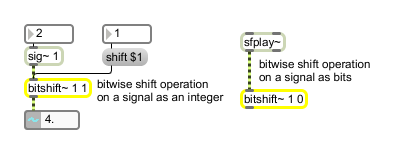bitshift~

Bit shifting for floating point signals

Description

bitshift~ performs bitwise operations on a floating point signal as bits or as an integer. Note that if you are converting the floats to ints and back that single precision floating point values only retain 24 bits of integer resolution. The floating point bits from left to right are <1 sign bit> <8 exponent bits> <23 mantissa bits>. Positive shift values correspond to left shifting that number of bits, negative shift values correspond to right shifting that number of bits.

Arguments

Name Type Opt Description
number-of-bits/direction-of-shift int opt Sets the number of bits to be shifted on the incoming floating-point signal. Positive shift values correspond to left shifting that number of bits, negative shift values correspond to right shifting that number of bits.
operational-mode (0 or 1) int opt Specifies whether the floating signal or floating-point value will be processed as a raw 32-bit floating-point value or converted to an integer value for bit shifting. The modes of operation are:
Mode: Description:
0 int Treat floating-point signal input as a raw 32-bit value (default).
1 int Convert the floating-point signal input to an integer value.

Messages

 int operational-mode (0 or 1) [int] Sets the operational mode of the bitshift~ object as described by the mode message. float operational-mode (0 or 1) [float] Performs the same function as int. mode operational-mode (0 or 1) [int] In left inlet: The word mode, followed by a zero or one, specifies whether the floating signal or floating-point value will be processed as a raw 32-bit floating-point value or converted to an integer value for bit shifting. The modes of operation are: Mode Descriptions: 0 - Treat floating-point signal input as a raw 32-bit value (default). 1 - Convert the floating-point signal input to an integer value. Note: If you convert the floating-point signal input to an int and then convert it back, the resulting floating-point value will retain only 24 bits of integer resolution. shift number-of-bits/direction-of-shift [int] In left inlet: The word shift, followed by a positive or negative number, specifies the number of bits to be shifted on the incoming floating-point signal. Positive number values correspond to left shifting that number of bits (i.e., Left shifting a number n places is the same as dividing it by 2n). Negative numbers correspond to right shifting that number of bits (i.e., Right shifting a number n places is the same as dividing it by 2n). signal The bitshift~ object performs bit shifting on a floating-point signal as either raw 32-bit data or as an integer value. Floating-point signal bit values are expressed in the following form: <1 sign bit> <8 exponent bits> <23 mantissa bits>

Output

signal: The resulting bit shifted floating-point signal.

Examples